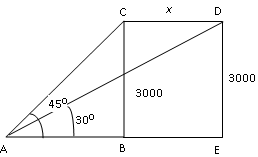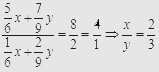Breaking News
Home / Placement Papers / Fidelity / Sample Aptitude Questions Of Fidelity

# Sample Aptitude Questions Of Fidelity

## Sample Aptitude Questions1.A Chartered Accountant applies for a job in two firms X & Y. The probability of his being selected in firm X is 0.7, and being rejected at Y is 0.5 and the probability of rejection at least one of his applications is 0.6. What is the probability that he will be selected in one of the firms?

A.0.8
B.0.2
C.0.4
D.0.7

P(X) = 0.7, P(Y) = 0.5 and P(X &union; Y) = 0.4. Therefore, P(X &intersection; Y) = P(X) + P(Y) – P(X &intersection; Y) = 0.7 + 0.5 – 0.4 = 0.8

2.Two small circular parks of diameters 16 m, 12 m are to be replaced by a bigger circular park. What would be the radius of this new park, if the new park has to occupy the same space as the two small parks?

A.15m
B.10m
C.20m
D.25m

π(8)2 + π(6)2 = πR2 => R = 10

3.A conical vessel of base radius 2 cm and height 3 cm is filled with kerosene. This liquid leaks through a hole in the bottom and collects in a cylindrical jar of radius 2 cm. The kerosene level in the jar is

A.π cm
B.1.5 cm
C.1 cm
D.3 cm

Let the level of kerosene in the cylinder = H.
volume of cone = volume of the cylinder
=> 1/3π(2)2(3) = π(2)2H => H = 1 cm

4.The angle of elevation of an aeroplane from a point on the ground is 45°. After 15 seconds flight, the elevation changes to 30°. If the aeroplane is flying at a height of 3000 m, the speed of the plane in km per hour is

A.208.34
B.306.72
C.402.56
D.527Let the plane at the start is at point C and after 15 seconds it reaches point D after covering x km as shown in diagram.
In Δ ABC, tan 45° = BC/AB => 1 = BC/AB => AB = BC = 3,000.
In %Delta; ADE, tan 30°= ED/(AB+BE) => 3000/(3000+BE) => BE = 2196 m .
Therefore x = 2.196 km.
So aeroplane covers 2.196 km in 15 seconds, so speed of aeroplane = (2.196 x 60 x 60)/15 = 527kmph.

5.At the first stop on his route, a driver unloaded 2/5 of the packages in his van. After he unloaded another three packages at his next stop, 1/2 of the original number of packages remained. How many packages were in the van before the first delivery?

A.25
B.10
C.30
D.36

Let the packages = P. Therefore, after first delivery he unloaded 2/5 of P and then he unloaded 3
more packages. So total packages unloaded = 2/5 of P + 3. According to the given condition
2/5 of P + 3 = 1/2 of P => P = 30

6.If S is 150 percent of T, then T is what percent of S + T?

A.40%
B.100/3%
C.75%
D.80%

S = 1.5 T. Now T/(S + T) x 100 = T/2.5T x 100 = 40%

7.An investor earns 3% return on 1/4th of his capital, 5% on 2/3rd and 11% on the remainder. What is the average rate of return he earns on his total capital?

A.10%
B.5%
C.5.5%
D.10.5%

Let the capital be C.
Therefore, 3% of 1/4 C + 5% of 2/3 C + 11% of (C – 1/4C – 2/3C) = R % of C => R = 5%

8.Mixture of milk and water has been kept in two separate containers. Ratio of milk to water in one of the containers is 5 : 1 and that in the other container is 7 : 2. In what ratio should the mixtures of these two containers be added together so that the quantity of milk in the new mixture may become 80%?

A.3 : 2
B.2 : 3
C.4 : 5
D.None of these

Let x litres of mixture be taken from container having 5 : 1 ratio and y litres be taken from 7 : 2 mixture container.
Therefore, according to the given condition,So they should be mixed in ratio 2 : 3

9.Rahul started a business with a capital of Rs. 8,000. After six months, Sanjay joined him with an investment of some capital. If at the end of the year each of them gets an equal amount as profit, how much did Sanjay invest in the business?

A.Rs. 17,500/-
B.Rs. 18,000/-
C.Rs. 16,000/-
D.Rs. 16,500/-

Rahul’s investment
= Capital × Time period = 8000 × 12. Sanjay’s Investment = C × 6.
As both of them share equal profit, so Rahul’s investment = Sanjay’s investment
=> 8000 × 12 = C × 6 => C = Rs. 16,000.

10.Sita buys a fridge at 15/16 of its original value & sells it for 10 % more than its value. Then gain % is

A.15.55
B.11.67
C.16.67
D.None of these